# 爱恨交织的红黑树ytao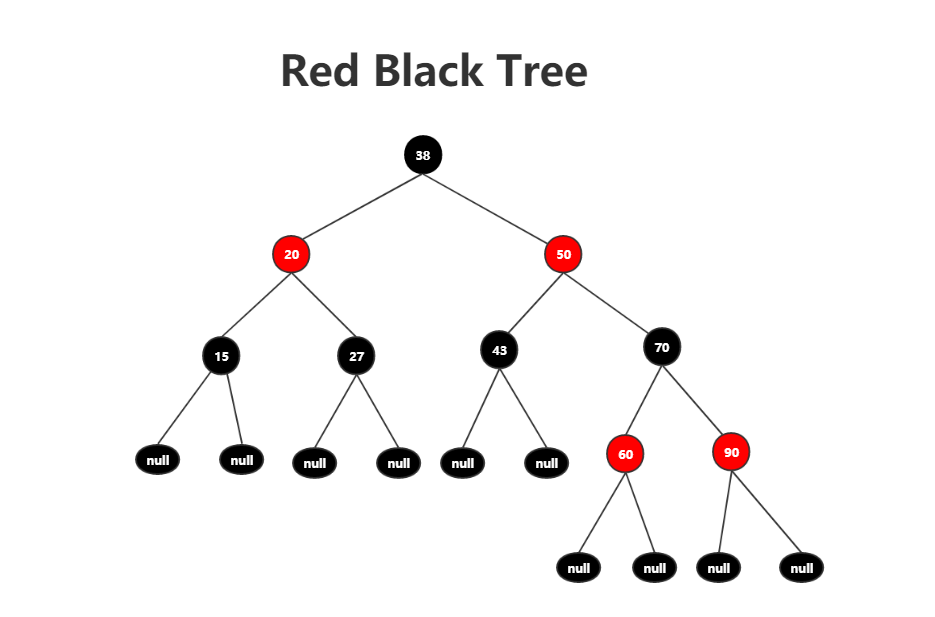# 特性

1. 结点是红色或黑色

2. 根结点必须为黑色

3. 叶子结点（约定为null）一定为黑色

4. 任一结点到叶子结点的每条路径上黑色结点数量都相等

5. 不允许连续两个结点都为红色，也就是说父结点和子结点不能都为红色

# 查找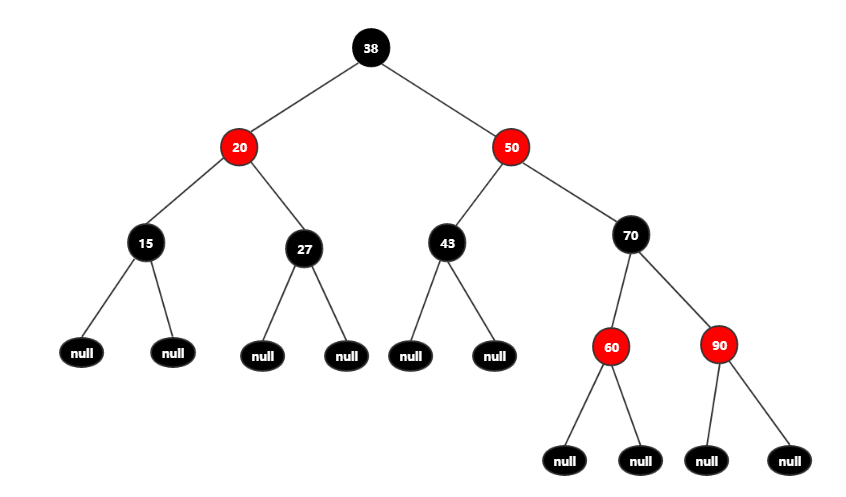# 普通操作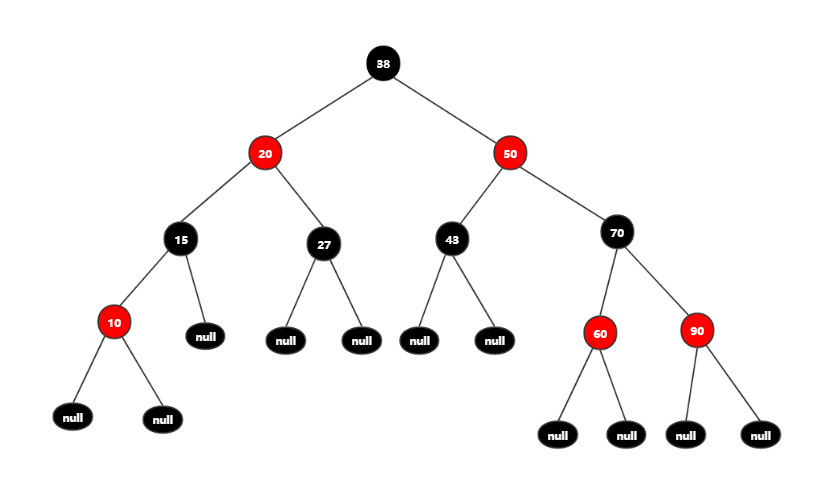package com.ytao.rbt;/** * Created by YANGTAO on 2019/11/9 0009. */public class Node {    public static String RED = "red";    public static String BLACK = "black";    public Integer value;    public String color;    public Node left;    public Node right;    public Node(Integer value, String color, Node left, Node right) {        this.value = value;        this.color = color;        this.left = left;        this.right = right;    }    public Node(int value, String color) {        this.value = value;        this.color = color;    }}

public void commonInsert(Node node, Integer newVal){    if (node == null)        node = new Node(newVal, Node.BLACK);        while (true){        if (newVal < node.value){            if (node.left == null){                // 如果左树为叶子结点并且父结点为黑色，可以直接插入红色新结点                if (node.color == Node.BLACK){                    node.left = new Node(newVal, Node.RED);                    break;                }            }            node = node.left;        }else if (newVal > node.value){            if (node.right == null){                if (node.color == Node.BLACK){                    node.right = new Node(newVal, Node.RED);                    break;                }                            }            node = node.right;        }    }}

# 变色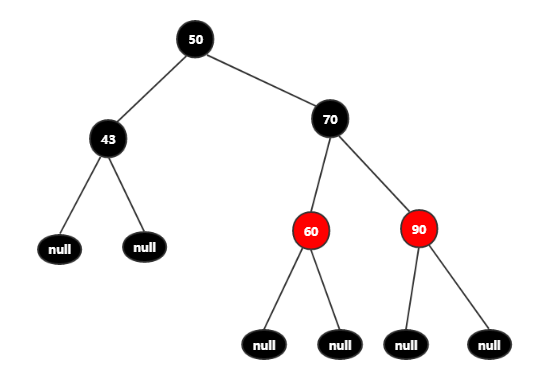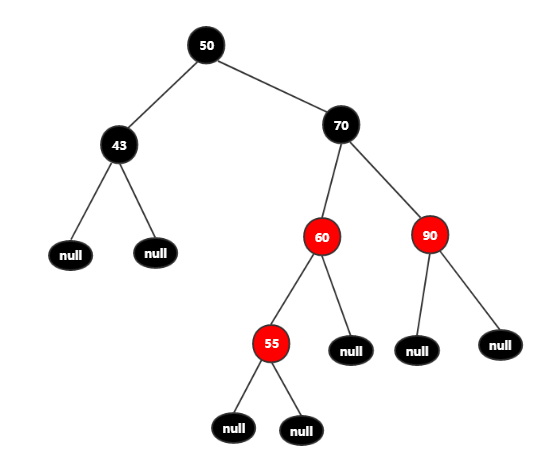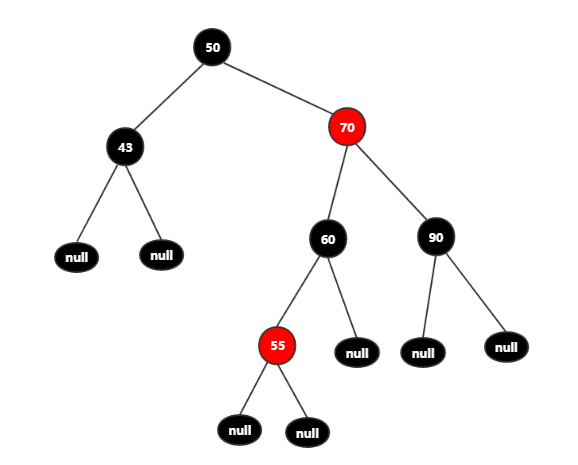public void changeColor(Node node, int newVal){    if (node.left == null || node.right == null)        return;    // 通过判断待插入结点的父结点和叔叔结点，是否满足我们需要的条件    if (node.left.color == Node.RED && node.right.color == Node.RED){        // 确定是更新到左树还是右树中        Node base = compare(newVal, node.value) > 0 ? node.right : node.left;        // 和待插入结点的父结点作比较        if (newVal < base.value && base.left == null){            base.left = new Node(newVal, Node.RED);        }else if (newVal > base.value && base.right == null){            base.right = new Node(newVal, Node.RED);        }    }    node.color = Node.RED;    // 通过取反获取插入结点的叔叔结点并将颜色变黑色    Node uncleNode = compare(newVal, node.value) > 0 ? node.left : node.right;    uncleNode.color = Node.BLACK;}public int compare(int o1, int o2){    if (o1 == o2)        return 0;    return o1 > o2 ? 1 : -1;}

# 旋转

## 左旋转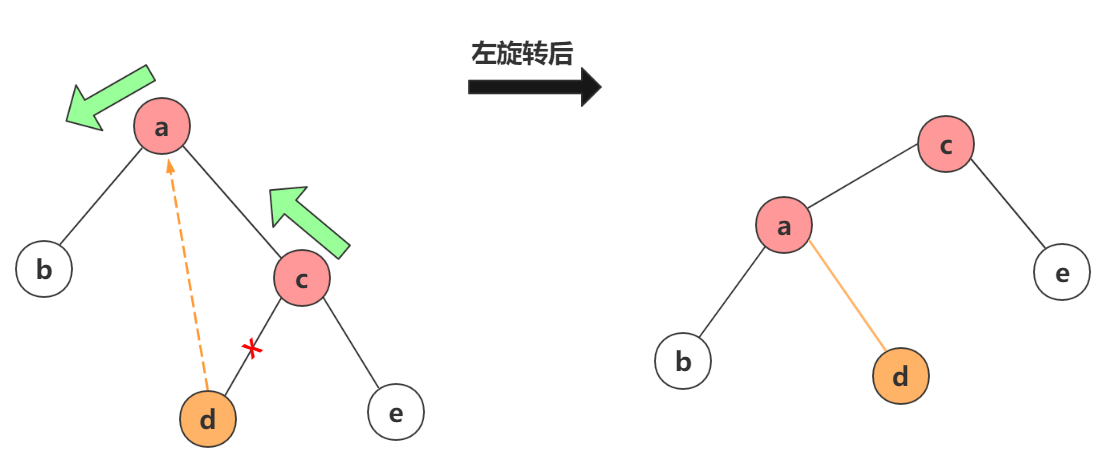## 右旋转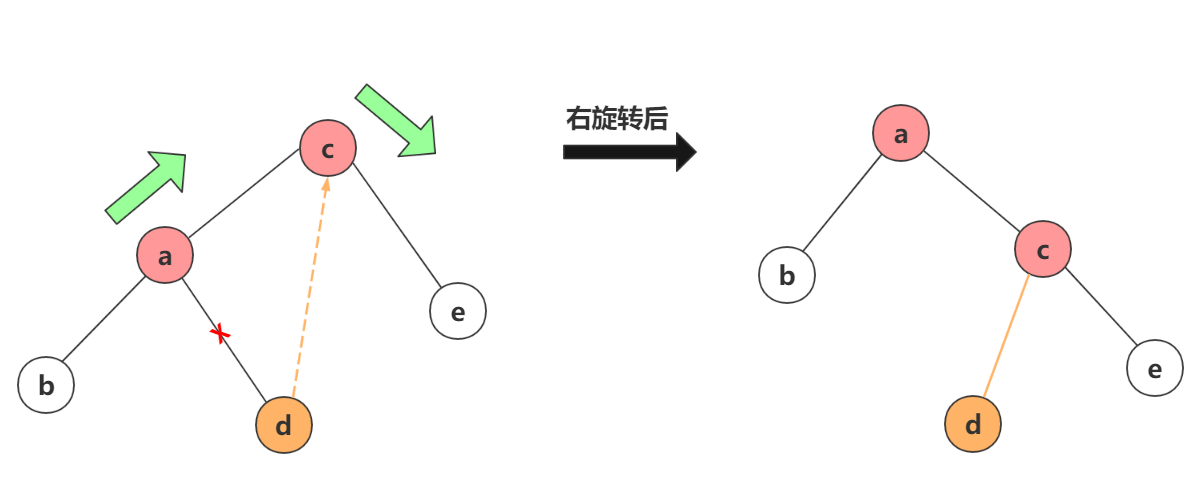/** * * @param node 两个旋转结点中的父结点 * @param value 两个旋转结点中子结点的值，因为在整合旋转的时候，node可以遍历查找出来，value作为需要旋转的标记结点 */public void rotate(Node node, int value){    Node nodeChild = compare(value, node.value) > 0 ? node.right : node.left;    if (nodeChild != null && value == nodeChild.value){        Node parent = node;        // 旋转子结点小于旋转父结点，执行的是右旋转，否则为左旋转        if (value < node.value){            rightRotate(parent);        }else if (value > node.value){            leftRotate(parent);        }    }}/** * 左旋转 * @param node 旋转的父结点 */public void leftRotate(Node node){    Node rightNode = node.right;    // 旋转结点的左子结点给父结点的右子结点    node.right = rightNode.left;    // 父结点作为子结点的左子结点    rightNode.left = node;}/** * 右旋转 * @param node */public void rightRotate(Node node){    Node leftNode = node.left;    // 旋转结点的右子结点给父结点的左子结点    node.left = leftNode.right;    // 父结点作为子结点的右子结点    leftNode.right = node;}

# 旋转变色案例应用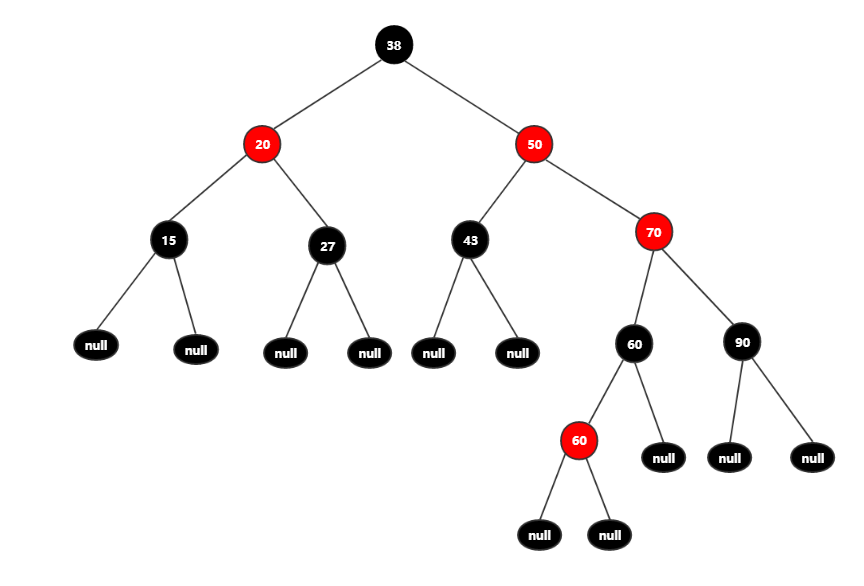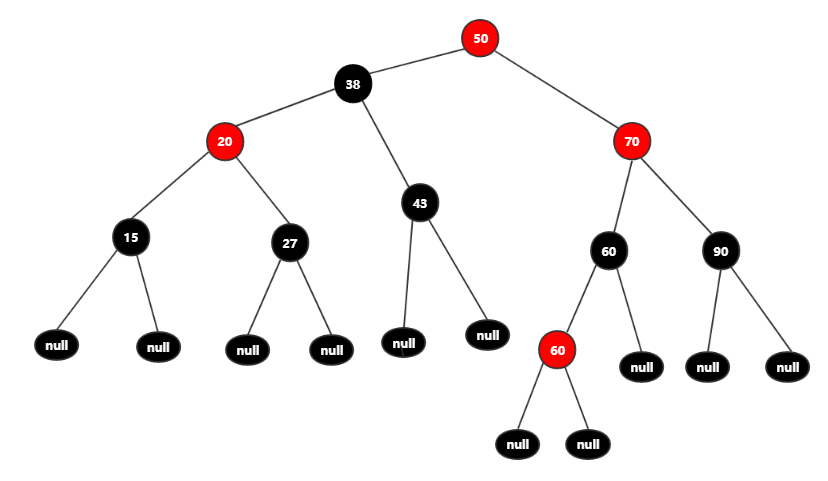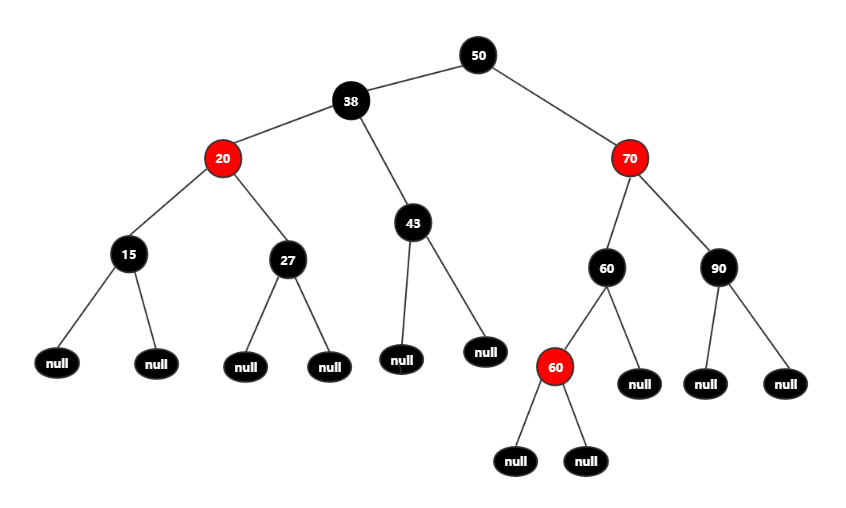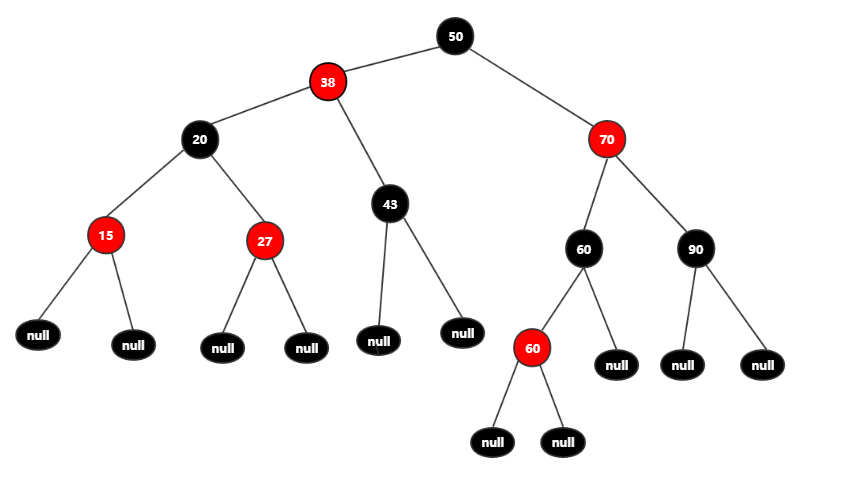# 总结

### 推荐阅读

《Redis5新特性Streams作消息队列》

《Netty中粘包/拆包处理》

《ElasticSearch之安装及基本操作API》### ytao

Java 开发 2018.05.03 加入

## 评论